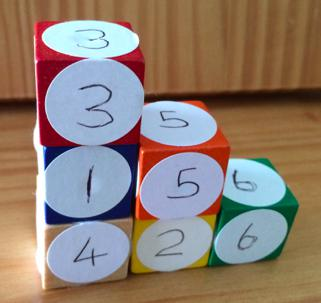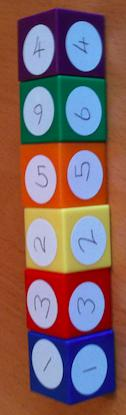#### You may also like### Cubes

How many faces can you see when you arrange these three cubes in different ways?### Pebbles

Place four pebbles on the sand in the form of a square. Keep adding as few pebbles as necessary to double the area. How many extra pebbles are added each time?### Bracelets

Investigate the different shaped bracelets you could make from 18 different spherical beads. How do they compare if you use 24 beads?

# Six Numbered Cubes

## Six Numbered Cubes

The aim of this challenge is to find the total of all the visible numbers on the cubes.

We are using six cubes.
Each cube has six faces of the same number.
.The "wall" has to be only one cube thick.
The one on the left is built correctly by being a wall only one cube thick.
The one on the right is NOT allowed as it is two bricks thick in parts.
The cubes sit neatly - square face against square face.
The total on the left one is 70.

CHALLENGE 1

Start by making a staircase shape.  An example is shown below:a/ What is the highest total you can make by using this staircase shape?

b/ What is the lowest total you can make by using this staircase shape?

c/ Explain in writing how you calculated the totals for a & b above, making sure you give reasons for your method/s.

d/ Now make a total of 75 using a staircase shape.

CHALLENGE 2

Using any shape of single cube thickness, what is the lowest total you can make?
How can you be sure this is the lowest total whatever the shape?
Can the lowest total be found in more than one way? Justify your answer.

CHALLENGE 3

Using any shape of single cube thickness, what is the highest total you can make?
How can you be sure this is the highest total whatever the shape?
Can the highest total be found in more than one way? Justify your answer.

CHALLENGE 4

Prove the following by logical reasoning, rather than by calculating the answers:

If the cubes are arranged in a single vertical tower (like this)then whatever the order of cubes you cannot produce a total of 80.

### Why do this problem?

This problem gives pupils the opportunity to use knowledge and skills associated with spatial awareness, addition and multiplication, and to explain their thinking. It also involves keeping to rules that must be followed. The further they progress through the activity, the greater the opportunities for learners to use a whole variety of problem-solving skills. The activity also opens out the possibility of pupils asking “I wonder what would happen if . . .?”
Pupils’ curiosity may be easily aroused while trying to find solutions to the challenges.

### Possible approach

It would be good to demonstrate the kind of arrangements that are allowed as well as making those that break the rules for the pupils to decide on what is ok.

You may decide that you want the pupils to work in groups of three or four.  One set of numbered cubes will be needed for each group. Having set them the first challenge, it may be sufficient to stop there.  Challenges 2, 3 and 4 can be introduced straightaway or left to another occasion.

It is worthwhile noting that in the "steps" arrangement as shown on the problem page, the 5 and 6 both have four faces showing; the 1 and 4 both have three faces showing. So, the cubes that have the same number of faces showing can be swapped allowing for more arrangements to be possible.

You may find this copy of the task useful to print off and give to learners: SixNumberedCubes.pdf

### Key questions

How are you working out the totals?
How have you got to this arrangement?